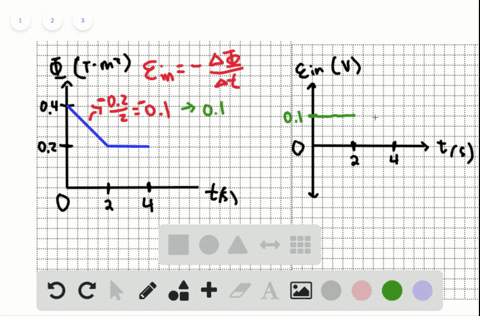🎉 The Study-to-Win Winning Ticket number has been announced! Go to your Tickets dashboard to see if you won! 🎉View Winning Ticket### The magnetic flux through three different coils i…

05:24Indian Institute of Technology Kharagpur
Problem 17

# The magnetic flux through three different coils is changing as shown in Figure $P 18.17$ . For each situation, draw a corresponding graph showing qualitatively how the induced emf changes with time

## Discussion

You must be signed in to discuss.

## Video Transcript

so the flat sources time graph is given So forest we will try to find defy DT With respect to time on, we can see that this is ah straight line. The functional form is phi equals negative Fine art. Thus some constant times t So if you differentiate it, then you will get a straight line and see And that we just make some space over here. We're trying to find the PMF induced, which is negative. Leafy DT. So this will be a straight line at a value off negative some constant on thesis is my part A Let's do part, B. Now this looks something like this and it looks like a parabola and the functional form can be recognized. FYI, eat grows she not. This is my feet. Not in the y axis. This is my firaxis minus C. He squared. So if I differentiate this function over here, I get dif e d t equals negative two c t. So this is a shred line with a negative slope which will look like something like this At Peak was Zito. I start from zero. No. Yeah. Man equals this and the slope is given by do you see over here? Part C part C. It will be difficult to write fee in ah functional form. However, I can see that this is a symmetry. Cars about the time axes that's going this up a little bit. That is my time axis. Well, we're here. Fox is constant. Then it linearly comes down and then it becomes costing again. That's a negative value. So once again, I'm going to find defeated ET, which is so over here you should understand that there is one section up to here where flux is not changing. So until that I have got my genuine starts to be zero from here onwards, once again, I have got my changing stuff to be zero. This will most likely be done better with covered graph. So this just draw the time axis to start wheat on and up until this section, I will have by changing starts to be zero. From here on the wars, I will have checking stops to be zero de fi dd Ah, he's the delivery of this lie which will have some negative value over here and now. My electro motive force will come out of the here, here and here for all these cases. What I have done these I am used the formula where equals negative. Do you fee DT?